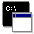## Firefly and PC GAMESS-related discussion club

Learn how to ask questions correctlyRe^2: Energy rises and oscillate at the end of optimization

Oleg Levitskiy
levicoleg@mail.ru

Dear Pavlo,
optimization with corrected parameters did 9 steps and unfortunately almost all of them with energy encrease. It seems that convergence to the predefined gradient value can't be achieved in case of applying solvent modeling as you've supposed...
Nonetheless I attached the resulting out in hope of appearing of any new ideas.

Thanks again,

Oleg

On Thu Sep 11 '14 5:18am, Pavlo Solntsev wrote
----------------------------------------------
>I would recommend you try these parameters first:
> \$contrl scftyp=rhf dfttyp=pbe96
> runtyp=OPTIMIZE icharg=-1 nprint=-5
> maxit=100 ICUT=11ĀĀĀĀĀ ITOL=30 NZVAR=168 \$end
> \$system mwords=100 \$end
> \$SCF DIRSCF=.true. maxdii=20 \$end
> \$ZMAT DLC=.T. AUTO=.T.\$end
> \$p2p p2p=.t. dlb=.t. \$end
> \$basis gbasis=TZV \$end
> \$PCM PCMTYP=DPCM SOLVNT=input RSOLV=2.155 EPS=35.688 EPSINF=1.806874 \$END
> \$PCMCAV RIN(58)=1.956 \$END

>Before apply a solvent correction, you need to optimize the geometry in a gas phase. Make sure you use a right functional. I am skeptical about pbe96. Do not expect very tight gradient for the geometry with the solvent correction. Usually 10^-4 is ok.

>Pavlo.
>
>
>
>On Tue Sep 9 '14 3:30pm, Oleg Levitskiy wrote
>---------------------------------------------
>>Dear Firefly Users,
>>I've tryed to perform an optimization of anionic complex of Ni (DFT/PBE96, PCM) and faced a problem with convergence. Energy has unexpectedly risen and fluctuate spontaneousely from step to step. I've tryed to increase accuracy (ICUT, ITOL values and also DLCTOL and ORTTOL), to change optimization method (GDIIS, NR), to perform optimization in cartesian or delocalized coordinates, but the result was similar in all cases. Could you advise me something else to solve this problem.
>>The beginning of my input is following:

>> \$contrl scftyp=rhf dfttyp=pbe96
>>runtyp=OPTIMIZE icharg=-1 nprint=-5
>>maxit=100 ICUT=20 ITOL=30 NZVAR=168 \$end
>> \$system kdiag=-1 mwords=100 \$end
>> \$SCF DIRSCF=.true. maxdii=20 \$end
>> \$STATPT method=NR \$end
>> \$ZMAT DLC=.T. AUTO=.T.
>>DLCTOL=1D-7 ORTTOL=1D-7 \$end
>> \$INTGRL PACKAO=.true. \$end
>> \$p2p p2p=.t. dlb=.t. \$end
>> \$basis gbasis=TZV \$end
>> \$PCM PCMTYP=DPCM SOLVNT=input RSOLV=2.155 EPS=35.688 EPSINF=1.806874 \$END
>> \$PCMCAV RIN(58)=1.956 \$END

>>Typical out-file is attached.

>>Thank you,

>>Oleg
>>This message contains the 1688 kb attachment [ GlyNi_deprot_MeCN_3.out ]Thu Sep 11 '14 8:48pmThis message read 534 times